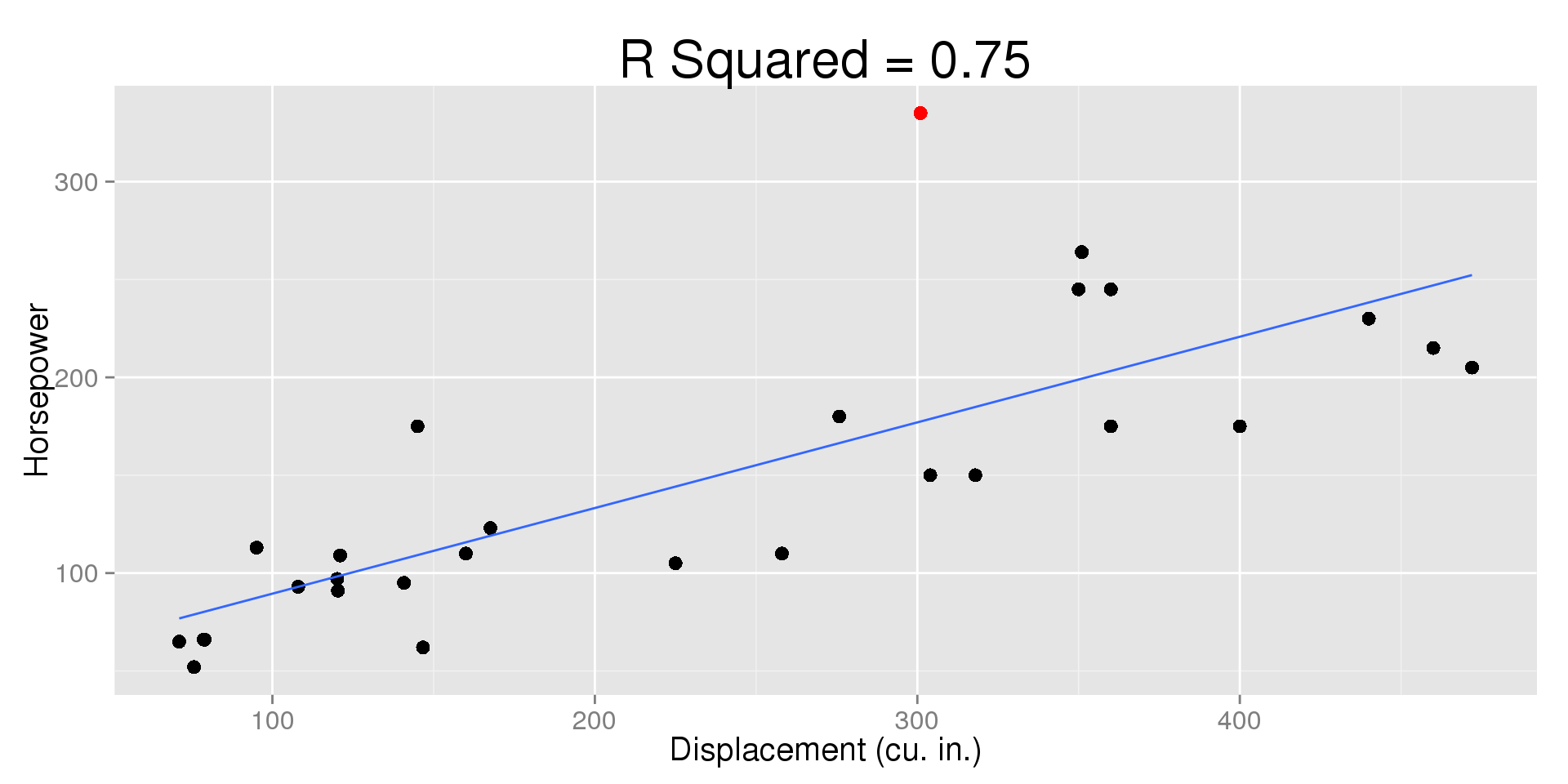5/27/2015

## Review

In Chapter 7, we saw:

• Scatterplots can show us relationships between two numeric variables.
• Correlation describes the strength and direction of linear relationships between two numeric variables.
• We call the $$X$$ variable the explanatory variable, because it explains the $$Y$$ variable.
• We call the $$Y$$ variable the response because it responds to changes in $$X$$.

Where do we go from here?

• If correlation tells us how well the points fit around a line, what line do they fit around?
• How can we use this line to describe the relationship further?
• Can we use it to make predictions?

## Fuel Efficiency vs. Weight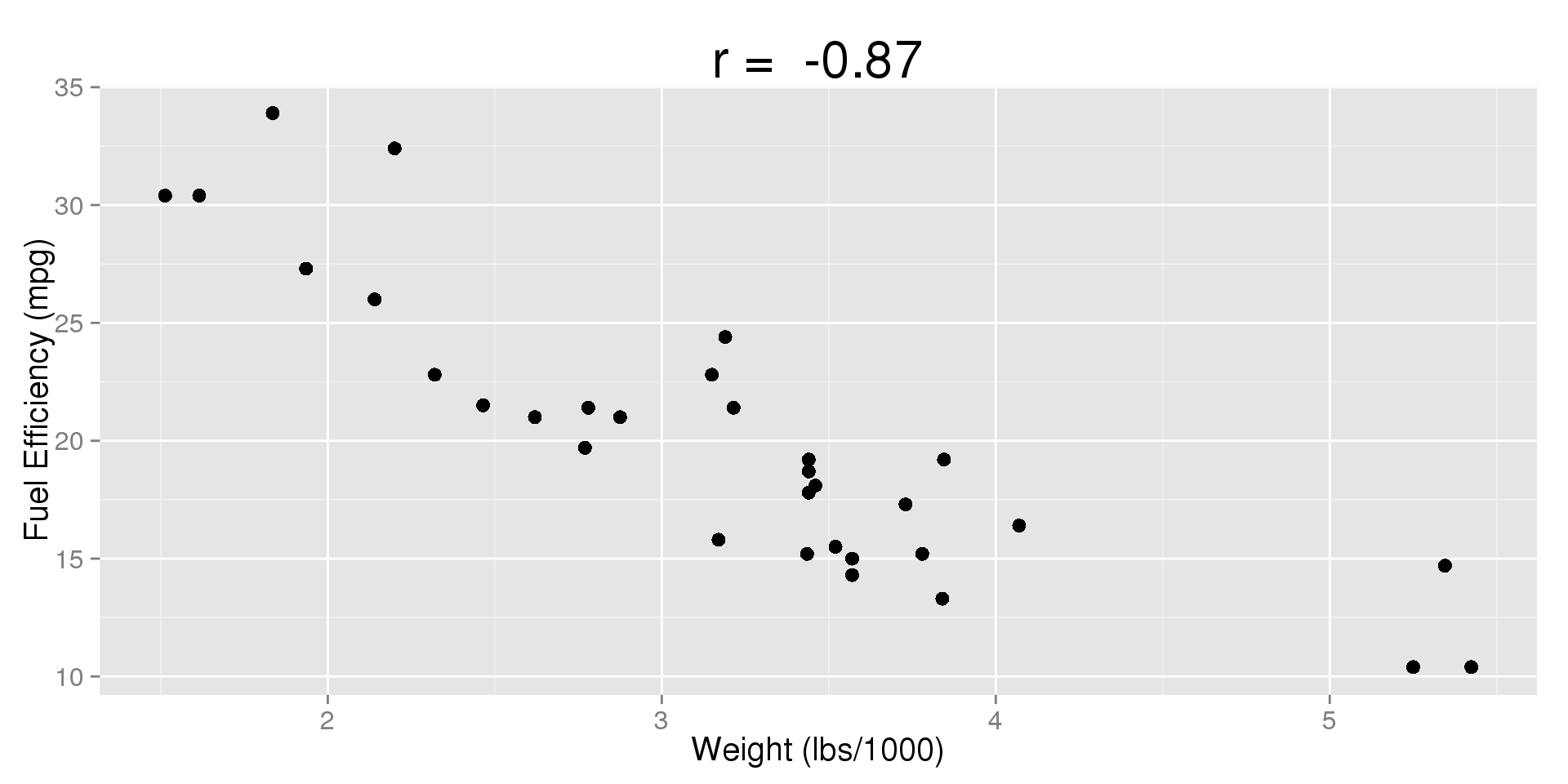## Fuel Efficiency vs. Weight

What can we see?

• $$r = -0.87$$
• There is a fairly strong negative linear relationship between the weight of a car and its fuel efficiency
• As we increase the weight of a car, the fuel efficiency tends to decrease.

What else might we want to do?

• Describe the general trend with a line
• Make a prediction of what the fuel efficiency of a car weighing 4500 lbs is.

## The Line of Best Fit

So how do we find the line the best describes the general trend?

• The most commonly used method is called the least squares regression (LSR).
• The least squares regression line is the line that comes closest to all of the points simultaneously.
• At any value of the $$X$$ variable, the line is our prediction for the mean or expected value of the $$Y$$ variable.
• This lets us analyze values of $$Y$$ given that we know what $$X$$ is.

## Fuel Efficiency vs. Weight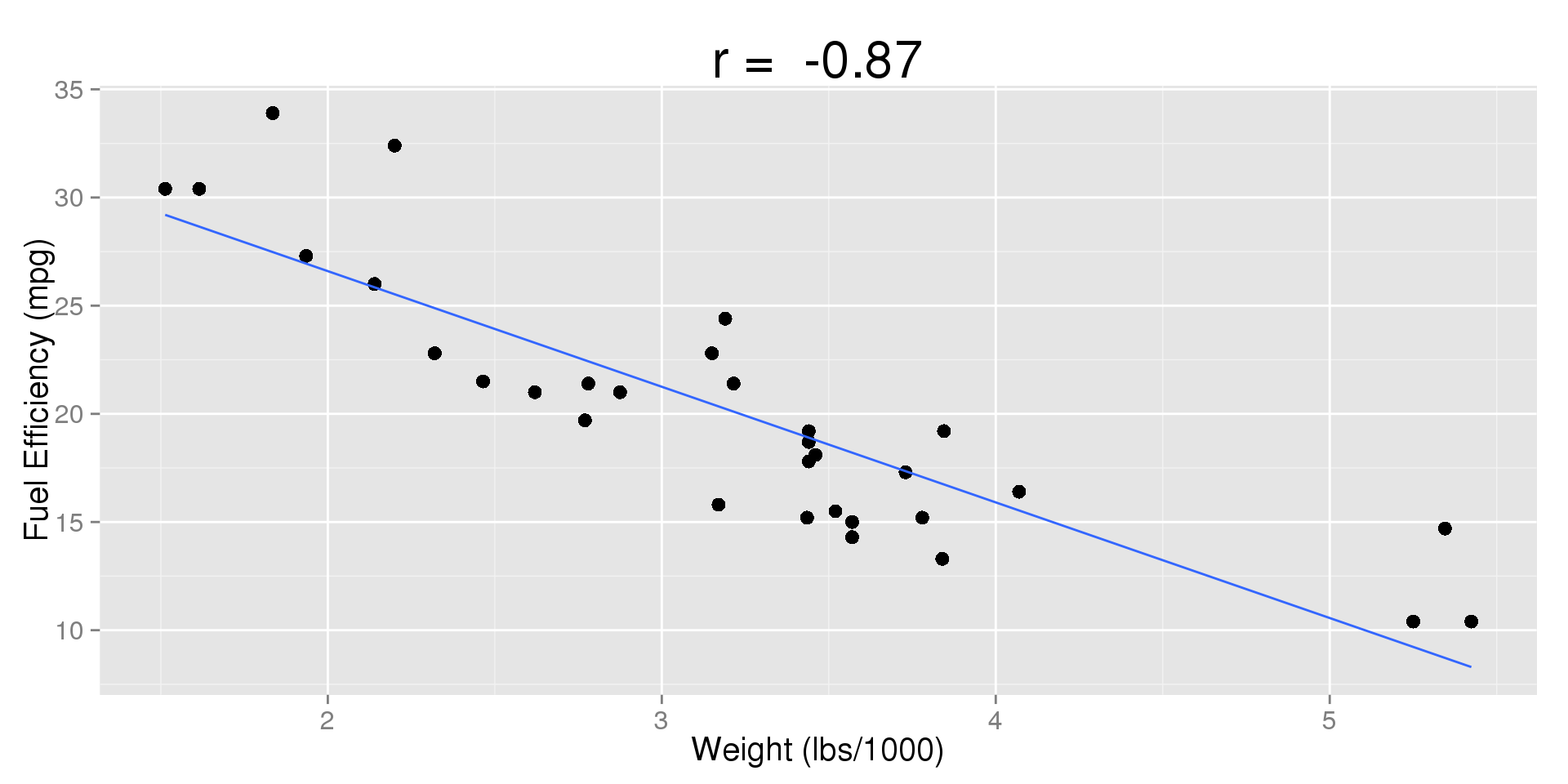## The Linear Model

Defining Least Squares Regression:

• LSR is a linear model
• A mathematical model is just a formula that describes something in the real world (e.g., Area = Base $$\times$$ Height)
• A statistical model does the same thing, but it accounts for variability or uncertainty (e.g., The Normal Model)
• LSR calculates the best possible line to describe the overall trend between our variables from our data

## The Least Squares Line

Recall from algebra that we can describe a line using the formula:

• $$y = mx + b$$

In statistics, we use the same method with different, but we label things differently:

• $$\hat{y} = b_0 + b_1 x$$

What do the terms mean?

• $$b_1$$ $$(m)$$ is the slope
• $$b_0$$ $$(b)$$ is the y-intercept
• $$\hat{y}$$ is our prediction for the mean of $$Y$$ when $$X = x$$

## Algebra Review: Lines

Consider the line: $$\hat{y} = 1 + 2x$$

$$x$$ $$\hat{y} = 1 + 2x$$ $$\hat{y}$$
$$0$$ $$\hat{y} = 1 + 2(0)$$ $$1$$
$$1$$ $$\hat{y} = 1 + 2(1)$$ $$3$$
$$2$$ $$\hat{y} = 1 + 2(2)$$ $$5$$

What do the coefficients tell us?

• $$b_0 = 1$$ tells us the value of $$\hat{y}$$ when $$X = 0$$
• $$b_1 = 2$$ tells us that every time $$X$$ goes up by one unit, $$\hat{y}$$ increases by $$2$$

## Fuel Efficiency vs. Weight

For our car data set, the regression line is:

$\widehat{mpg} = 37.2851 -5.3445wt$

What does this tell us? Keep in mind that weight is in thousands of pounds.

• For every added 1000 pounds of weight, fuel efficiency drops by $$5.3445$$ miles per gallon
• If a car weighs 0 lbs, it's predicted efficiency is $$37.285$$ mpg

This brings up an important note:

• A car can't weigh 0 lbs.
• In statistics, the y-intercept is often not interpretable, we just use it to draw the line and make predictions.

## Fuel Efficiency vs. Weight

$\widehat{mpg} = 37.2851 -5.3445wt$

If a car weighed $$4500$$ lbs, what would we expect its efficiency to be?

• $$\widehat{mpg} = 37.28511 -5.3445(4.5)$$
• $$\widehat{mpg} = 37.285 - 24.05$$
• $$\widehat{mpg} = 13.23$$

How do we interpret this?

• We predict that the average car weighing $$4500$$ lbs gets $$13.23$$ mpg

## Hitting Points

An important note:

• The Least Squares Regression line is not gauranteed to hit any one of our observed points
• In fact, it's entirely possible that the line will not hit any of our observed points
• There is only one place that we know the line will pass through: $$(\bar{x}, \bar{y})$$

## Hitting Points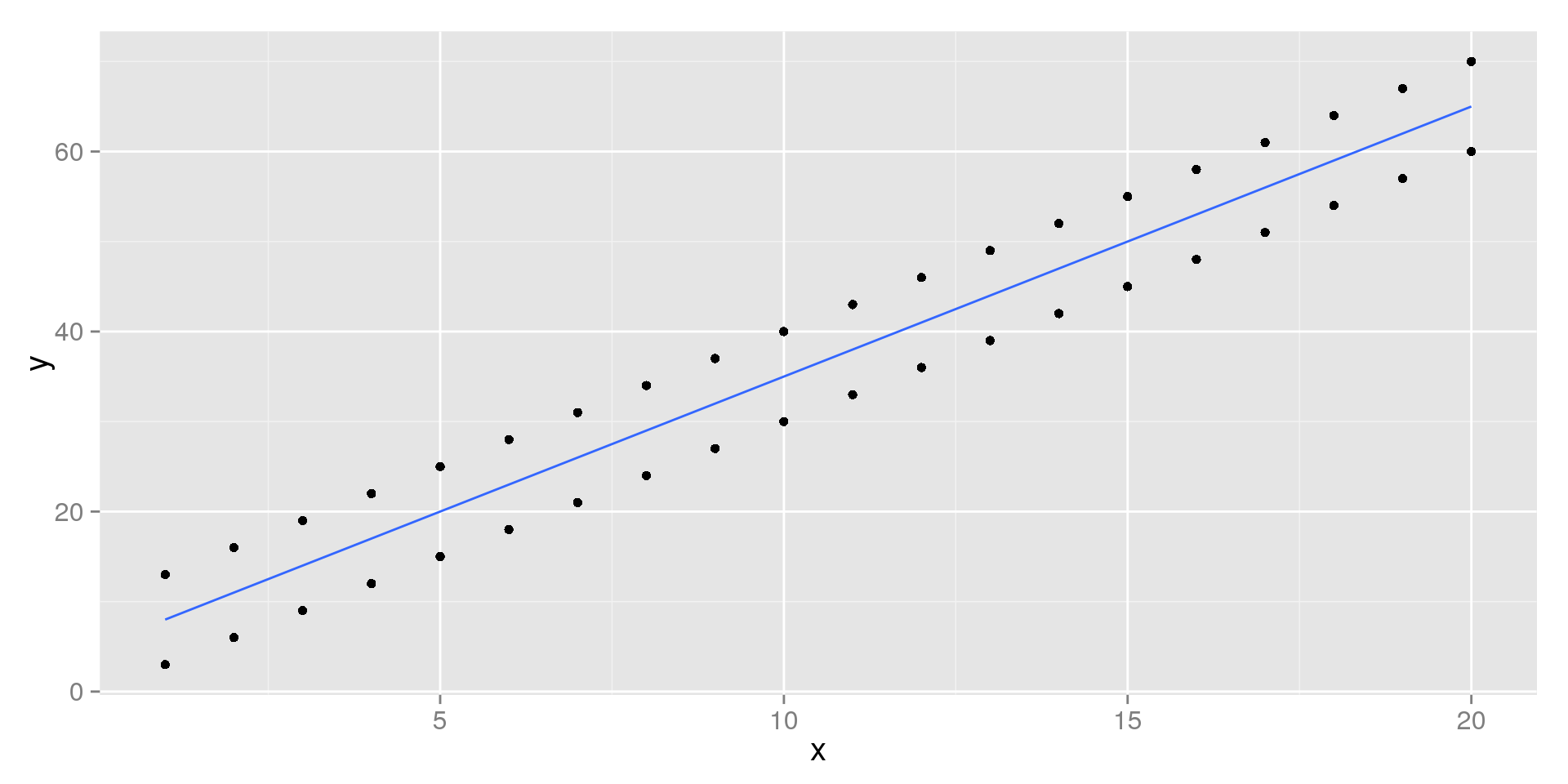## Residuals

We said the the Least Squares Regression line doesn't hit every point in our scatterplot, so how can we tell how well it does?

• For each point $$(x, y)$$, we predict the value $$(x, \hat{y})$$
• For every observation we have, we can see how far off we were by finding the residual
• For a given $$x$$, the residual is: $$y - \hat{y}$$
• This is the vertical distance between the line and the true value of $$y$$.
• If our estimate was too high, the residual will be negative
• If our estimate was too low, the residual will be positive

## Residuals: Example

Let's pick on car in our data set, the Camaro Z28. This car gets $$13.3$$ mpg and weighs $$3840$$ lbs.

• $$\widehat{mpg} = 37.2851 -5.34455wt$$
• $$\widehat{mpg} = 37.2851 -5.3445(3.84)$$
• $$\widehat{mpg} = 37.2851 - 20.5228$$
• $$\widehat{mpg} = 16.76$$

So how'd we do?

• $$mpg - \widehat{mpg} = 13.3 - 16.76 = -3.46$$
• We overestimated by 3.46 mpg

## Visualizing Residuals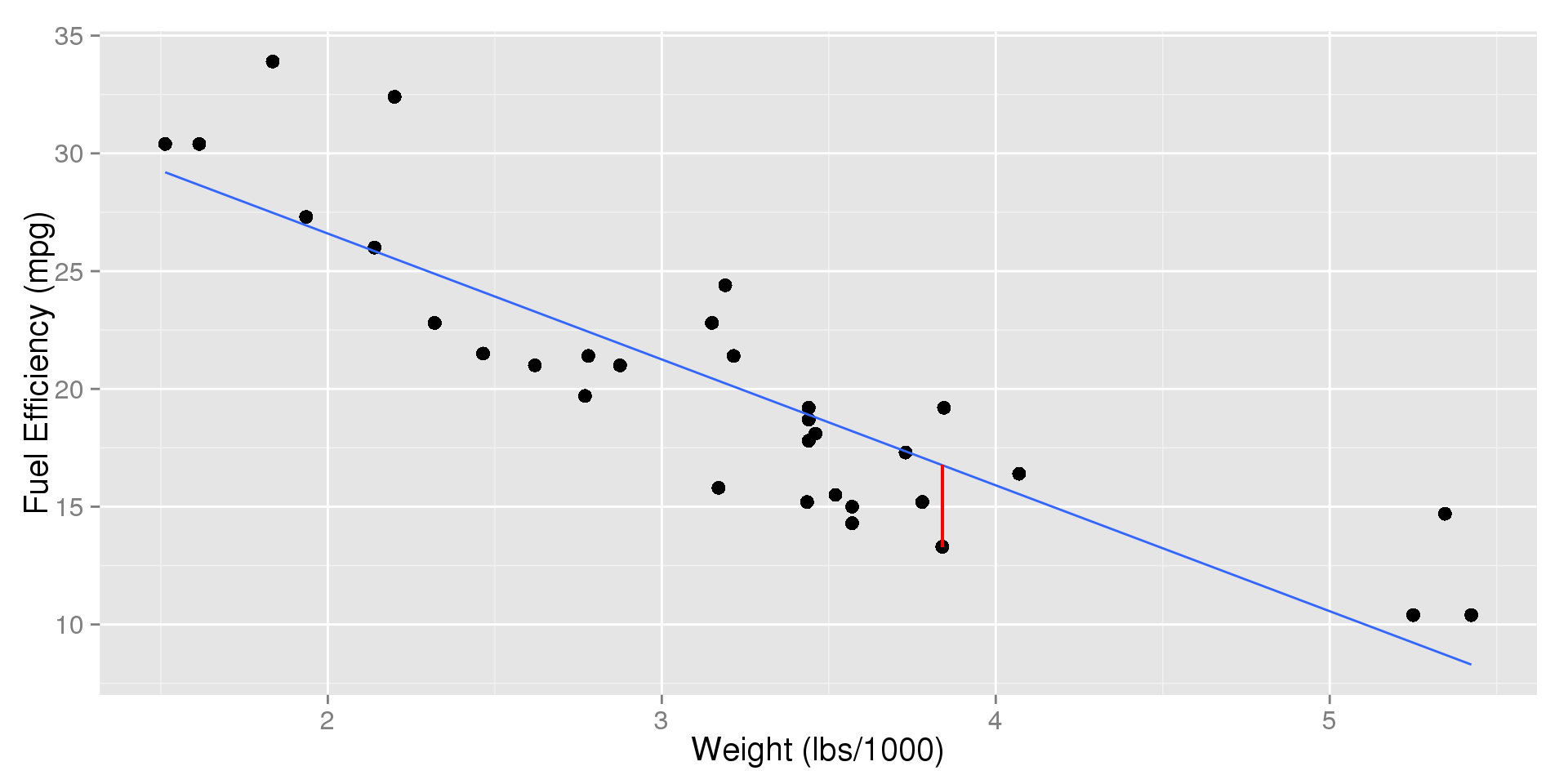## Residuals: Example

Let's pick another car, the Fiat 128. It's efficiency is $$32.4$$ mpg and it weights $$2200$$ lbs.

• $$\widehat{mpg} = 37.2851 -5.34455wt$$
• $$\widehat{mpg} = 37.2851 -5.3445(2.2)$$
• $$\widehat{mpg} = 37.2851 - 11.76$$
• $$\widehat{mpg} = 25.53$$

So how'd we do?

• $$mpg - \widehat{mpg} = 32.4 - 25.53 = 6.87$$
• The model underestimated by 6.87 mpg

## Visualizing Residuals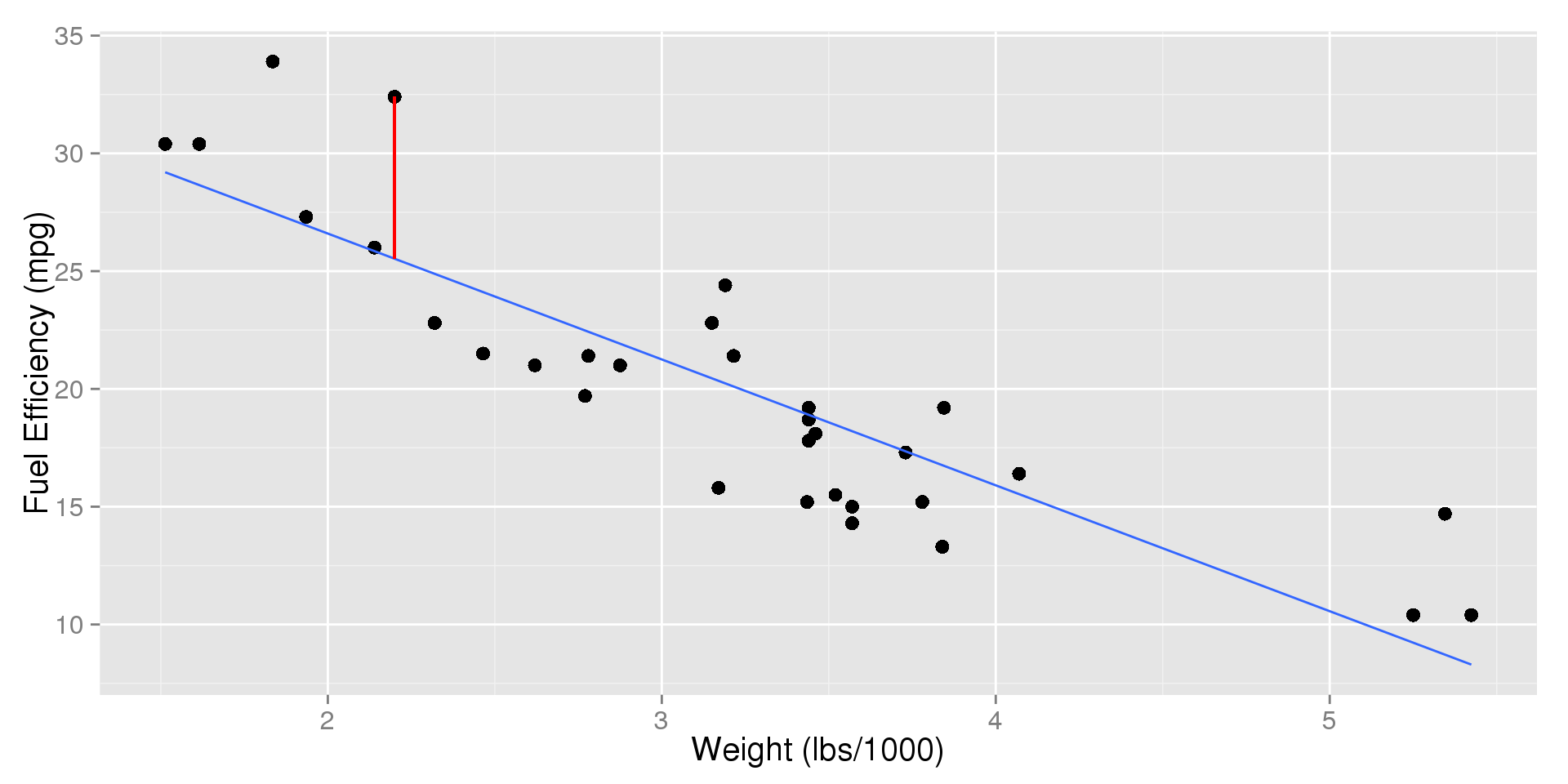## Residuals in Reverse

Imagine you bought a car that weighs $$2780$$ pounds, and I told you the residual was $$-1.03$$. What was the car's fuel efficiency?

• $$mpg - \widehat{mpg} = -1.03$$
• $$\widehat{mpg} = 37.2851 -5.34455wt$$
• $$\widehat{mpg} = 37.2851 -5.3445(2.78)$$
• $$\widehat{mpg} = 37.2851 - 14.86$$
• $$\widehat{mpg} = 22.43$$
• $$mpg - 22.43 = -1.03$$
• $$mpg = -1.03 + 22.43 = 21.4$$

## The Least Squares Line

We've seen how to check the prediction for a single value, but how do we know we did the best we could?

• The LSR line comes the closest to all of the points simultaneously
• It does this by finding the line which has the smallest residuals overall
• Since negative residuals are just as important as positive ones, we square them to force them to be positive
• Because of this, we focus on the squared residuals

We call our line the Least Squares Regression line because it:

• Minimizes the sum of the squared residuals
• This means that it minimizes the sum of the squared vertical distances between the points and the line.

## Relationship to Correlation

Recall:

• Correlation is the strength of the linear relationship between $$X$$ and $$Y$$.
• $$-1 \le r \le 1$$
• The direction of the relationship is indicated by the sign of $$r$$

Because of this,

• The sign of $$r$$ will always match the sign of $$b_1$$.

## Measure of Fit: $$R^2$$

In order to evaluate how good the model is, we need a measurement of how well it fits. For this, we use the $$R^2$$ statistic.

• $$R^2$$ is the fraction of the variability in the response $$(Y)$$ variable exlained by the $$X$$ variable.
• Do changes in $$X$$ explain changes in $$Y$$?

So what is $$R^2$$?

• If we only have one $$X$$ variable, $$R^2 = r^2$$
• $$-1 \le r \le 1 \quad \to \quad 0 \le R^2 \le 1$$
• If $$R^2 = 1$$, knowing $$X$$ lets us perfectly predict $$Y$$
• If $$R^2 = 0$$, $$X$$ tells us nothing about $$Y$$

## Fuel Efficiency vs. Weight

What is the $$R^2$$ of our model that predicts fuel efficiency from weight?

• $$r = -0.87$$, there is a strong negative correlation
• $$R^2 = (-0.87)^2$$
• $$R^2 = 0.76$$
• So the weight of cars explains 76% of the variability in their fuel efficiency
• This makes sense, obviously other properties (number of cylinders, transmission, design, etc.) will play a role in a car's fuel efficiency

## Units in Regression

In Correlation:

• The correlation coefficient has no units
• Changes in units (e.g., lbs $$\to$$ kg) had no effect on $$r$$

In Regression:

• The slope is "rise over run", or "change in $$Y$$ over change in $$X$$"
• The slope is measured in units of $$Y$$ over units of $$X$$
• Changing units can significantly change our line

## Fuel Efficiency vs. Weight: Changes in Units

So far, we've been measuring the weight in 1000s of pounds

• $$b_1 = -5.3445$$
• This represents how much the fuel efficiency in mpg changes when we increase weight by 1000 pounds

What if we represented the weight directly in pounds?

• The same relationship needs to exist, so changing the weight by 1000 pounds still needs to move mpg down by 5.3445
• In order for this to be true, the slope needs to be divided by 1000
• $$b_1 = -5.3445/1000 = -0.0053445$$
• So changing increasing the weight by a single pound decreases mpg by 0.0053445.

## Regression: Outliers

How do outliers affect regression?

If they're above or below the line (extreme in $$Y$$):

• They can affect the slope drastically
• They can decrease $$R^2$$

If they fall along the line, but are extreme in $$X$$

• They can inflate $$R^2$$ and make us think the relationship is stronger than it really is

## Fuel Efficiency vs. Weight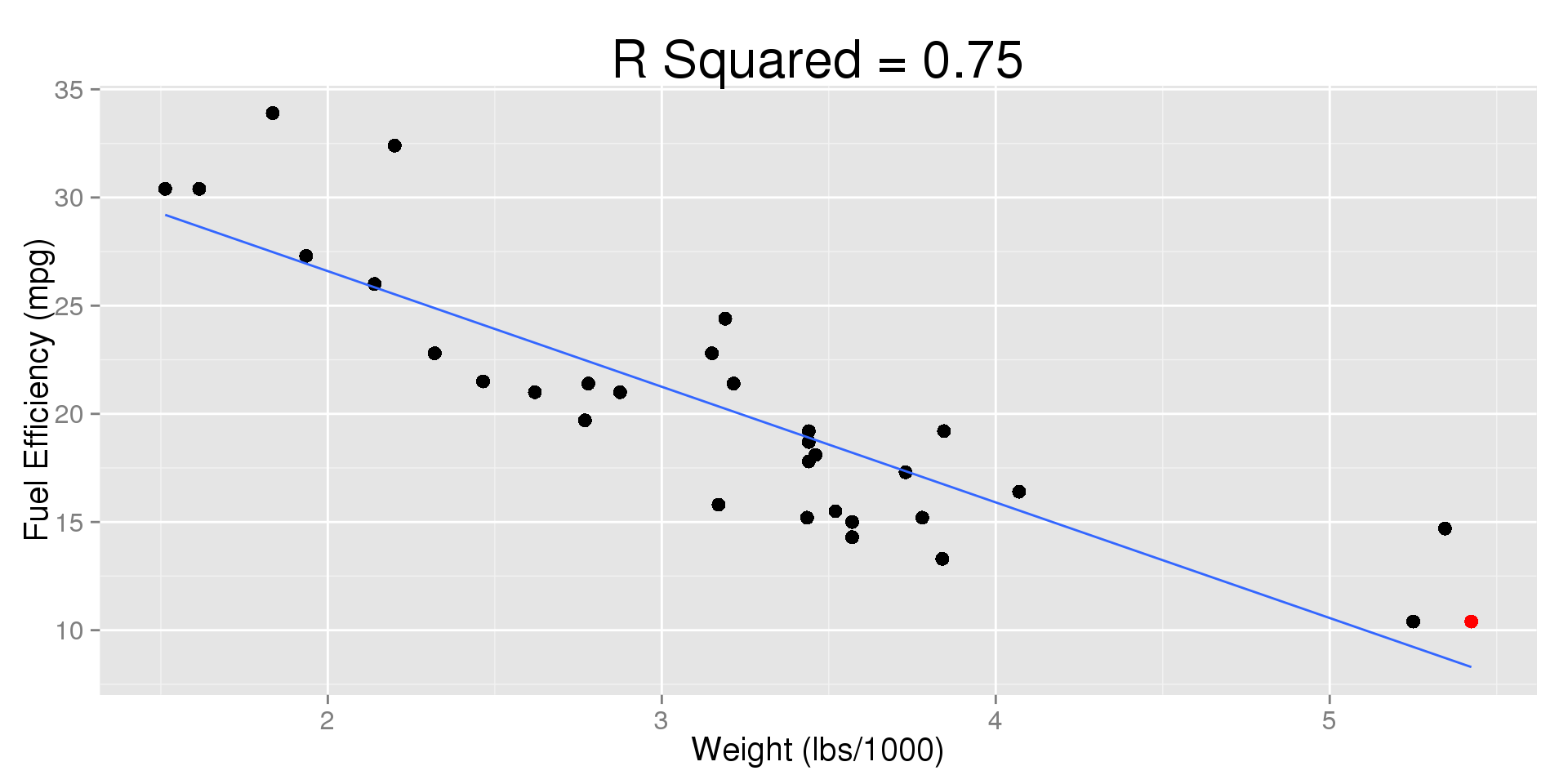## Fuel Efficiency vs. Weight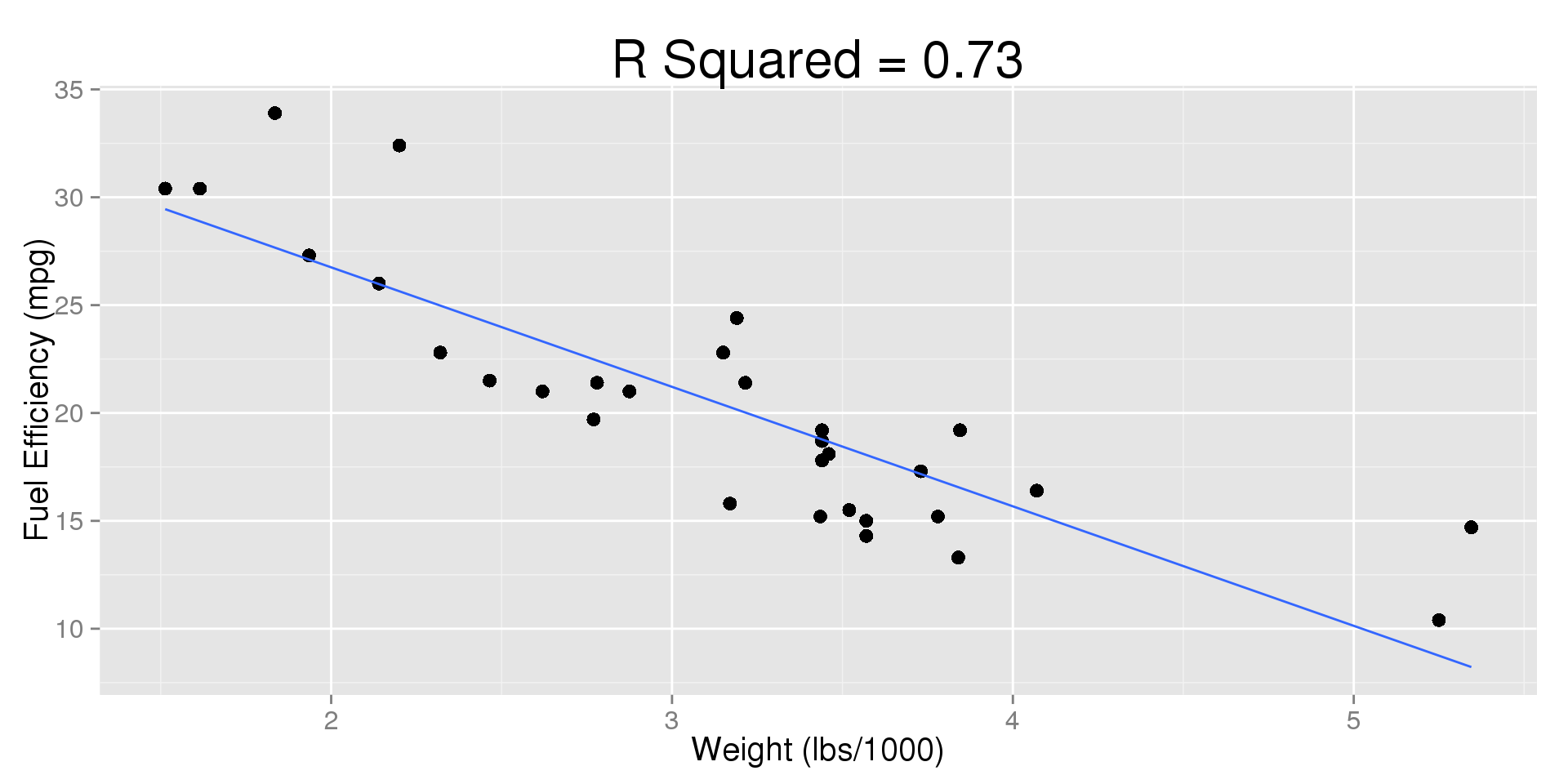## Horsepower vs. Engine Displacement